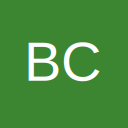# Test For/ Get Past Array "Subscript Out of Range" Error Message

• Hello,

I'm getting a Subcript Out of Range error for an array based on an Excel spreadsheet, and I can see that it is because there is a blank in the cell that the array is attempting to retrieve the element from. I have been trying to get past that error with no success.

For Example:

Code
``If cwlaArray(r, 3) = "" Then]``

returns "Subscript Out of Range" rather than True. I can't use an "On Error Resume Next" since I don't want the next lines of script executed.

Here is the part of my script where the problem occurs. The bolded line is where the error occurs. I would like it to exit the If and For statements.

Code
``````For r = 2 To UBound(cwlaArray, 1)
'Test whether the Customer Code in the CWLA File equals that in the Pyramid file
[B]If cwlaArray(r, 3) = PyrCC[/B] Then
ClientCode = cwlaArray(r, 1)
PyrRow = ecell.Row
Fname2 = "c:\Client Code Files\" & ClientCode & ".xlsx"
WriteRowtoClientFile
Exit For
End If
Next r``````

Any help is much appreciated.

Brian

• Re: Test For/ Get Past Array &quot;Subscript Out of Range&quot; Error Message

In that case you'd need to know the error number that was given with "subscript out of range" then you can check for the error number...etc

## Participate now!

Don’t have an account yet? Register yourself now and be a part of our community!